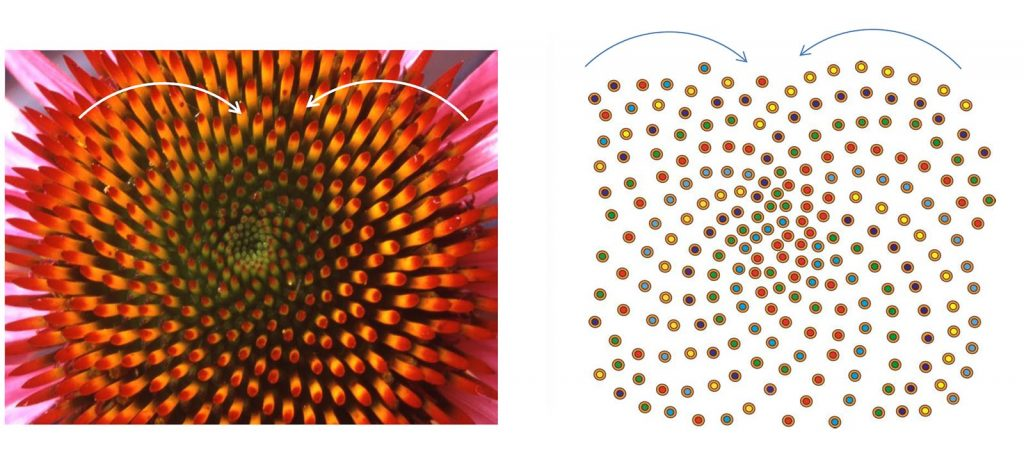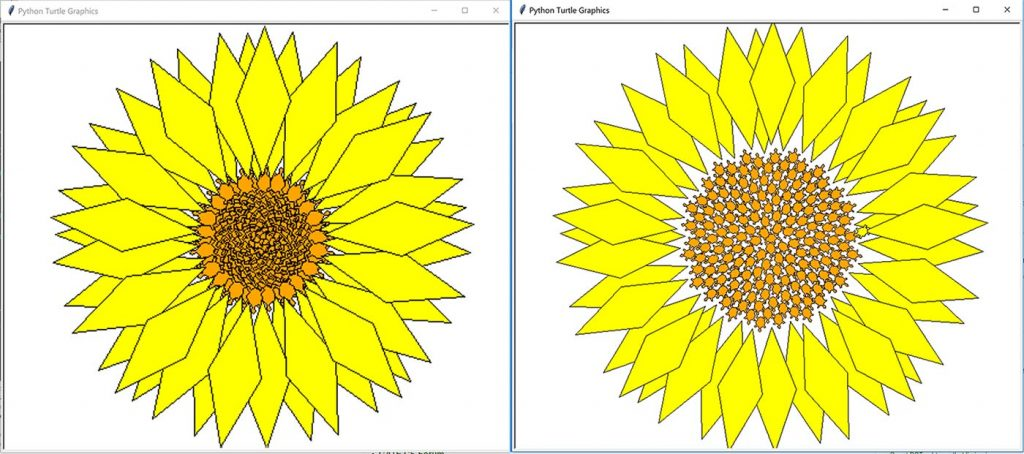# Phyllotaxis pattern in Python | A unit of Algorithmic Botany

• Last Updated : 07 Dec, 2020

Phyllotaxis/phyllotaxy is the arrangement of leaves on a plant stem & the Phyllotactic spirals form a distinctive class of patterns in nature. The word itself comes from the Greek phullon, meaning “leaf, ” and taxis, meaning “arrangement”.The basic floral phyllotaxic arrangements include:
1. Spiral Phyllotaxis – In spiral phyllotaxy, the individual floral organs are created in a regular time interval with the same divergent angle. The divergent angle in a flower with spiral phyllotaxy approximates 137.5 degrees, which is indicative of a pattern that follows a Fibonacci series.The image below shows the spiral phyllotaxy patterns having both clockwise and anticlockwise spiral patterns.Important points to note:

1. Fibonacci series typically describe spirals found in nature. It is calculated as a series where the previous pair of numbers sum to the next number in the series. The series is 1, 1, 2, 3, 5, 8, 13, 21, 34, 55, 89 … .
2. There is actually one set of spirals in a clockwise direction and one set in a counter-clockwise direction.
3. Floral organ spirals follow a numerator and denominator set of offset Fibonacci numbers (1/2, 1/3, 2/5, 3/8, 5/13, 8/21, 13/34 …). The numerator is the number of times or turns around the axis to get back to the initiation origin. The denominator indicates the number of organs initiated during the turns. Therefore, a 2/5 would indicate 2 turns around the axis and 5 organs to return to the origin.
4. e.g – In the pine we have (2, 3), (5, 3), and (5, 8) phyllotaxes, in capituli the pairs found are (21, 34), (55, 34), (55, 89), and (89, 144), and on pineapples with hexagonal scales the triplets (8, 13, 21) or (13, 21, 34) are found, depending on the size of the specimens .
5. The prevalence of the Fibonacci sequence in phyllotaxis is often referred to as “the mystery of phyllotaxis.”

Other types of floral phyllotaxic arrangements are:
2. Whorled Phyllotaxis, 3. Simple-whorled Phyllotaxis, 4. Complex-whorled Phyllotaxis & 5. Irregular Phyllotaxis

Formation of the Pattern : Summary

The beautiful arrangement of leaves in some plants, called phyllotaxis, obeys a number of subtle mathematical relationships. For instance, the florets in the head of a sunflower form two oppositely directed spirals: 55 of them clockwise and 34 counterclockwise. Surprisingly,

1. These numbers are consecutive Fibonacci numbers.
2. The ratios of alternate Fibonacci numbers are given by the convergents to φ^(-2), where φ is the golden ratio, and are said to measure the fraction of a turn between successive leaves on the stalk of a plant:
3. e.g : 1/2 for elm and linden, 1/3 for beech and hazel, 2/5 for oak and apple, 3/8 for poplar and rose, 5/13 for willow and almond, etc.
4. Each new leaf on a plant stem is positioned at a certain angle to the previous one and that this angle is constant between leaves: usually about 137.5 degrees.

That is, if you look down from above on the plant and measure the angle formed between a line drawn from the stem to the leaf and a corresponding line for the next leaf, you will find that there is generally a fixed angle, called the divergence angle.
Here, we are interested in Spiral phyllotaxy and we will code to form Spiral Phyllotaxy pattern in python using turtle graphics.

Designing the Code

1. We will code two functions, one to draw the phyllotaxy pattern and the other to draw the petals.
2. The petals need to be drawn only after the phyllotaxis pattern is completed.So, we will call the drawPetal() function from inside the drawPhyllPattern() function with the last x & y coordinates being visited after drawing the Phyllotaxis pattern.
3. The drawPetal() function will draw the petals with turtle functions and features, refer Turtle programming.

To code the phyllotaxis pattern, we need to follow these equations:

```x = r*cos(θ)
y = r*sin(θ)

r, θ can also vary - so the to form phyllotactic pattern we substitutethe cartesian form
by polar form:

r = c*sqrt(n)
θ = n*137.508°
``````Reduces the problem to optimal packing on a disc, so
r = c*sqrt(n) is from the area of the circle
Area = πr² and n fills the Area in some units
c1 * n/π = r²,    c is 1/sqrt(c1/π)
So, r = some constant c * sqrt(n)
```

PseudoCode : Phyllotaxis Pattern

```IMPORT MODULES ( MATH, TURTLE )

FUNCTION - DrawPhyllotaxisPattern( turtle, t length, petalstart, angle = 137.508, size, cspread)
turtleColor("Black")
FillColor('"Orange")
Convert angle to radians (Φ)
initialize ( xcenter,ycenter ) = ( 0,0 )
Drawing the Pattern Starts:
For n in Range ( 0,t ):
r = cspread * sqrt(n)
θ = n * Φ

x = r * cos(θ) + xcenter
y = r * sin(θ) + ycenter

TURTLE POSITION(x,y)
START DRAWING():
if Drawing pattern ends:
DrawFlowerPetals()

FUNCTION - DrawFlowerPetals(Turtle, x coordinate, y coordinate)
DRAW using Turtle methods

Create Turtle  =  gfg
Call DrawPhyllotaxisPattern( gfg, t length, petalstart, angle = 137.508, size, cspread)

END
```

## Python Pattern A

 `import` `math       ``import` `turtle`` ` `def` `drawPhyllPattern(turtle, t, petalstart, angle ``=` `137.508``, size ``=` `2``, cspread ``=` `4` `):``    ``"""print a pattern of circles using spiral phyllotactic data"""``    ``# initialize position``    ``# turtle.pen(outline=1, pencolor="black", fillcolor="orange")``    ``turtle.color(``'black'``)``    ``turtle.fillcolor(``"orange"``)``    ``phi ``=` `angle ``*` `( math.pi ``/` `180.0` `) ``#we convert to radian``    ``xcenter ``=` `0.0``    ``ycenter ``=` `0.0``    ` `    ``# for loops iterate in this case from the first value until < 4, so``    ``for` `n ``in` `range` `(``0``, t):``        ``r ``=` `cspread ``*` `math.sqrt(n)``        ``theta ``=` `n ``*` `phi``         ` `        ``x ``=` `r ``*` `math.cos(theta) ``+` `xcenter``        ``y ``=` `r ``*` `math.sin(theta) ``+` `ycenter`` ` `        ``# move the turtle to that position and draw ``        ``turtle.up()``        ``turtle.setpos(x, y)``        ``turtle.down()``        ``# orient the turtle correctly``        ``turtle.setheading(n ``*` `angle)``        ``if` `n > petalstart``-``1``:``            ``turtle.color(``"yellow"``)``            ``drawPetal(turtle, x, y)``        ``else``: turtle.stamp()``             ` ` ` `def` `drawPetal(turtle, x, y ):``    ``turtle.penup()``    ``turtle.goto(x, y)``    ``turtle.pendown()``    ``turtle.color(``'black'``)``    ``turtle.fillcolor(``'yellow'``)``    ``turtle.begin_fill()``    ``turtle.right(``20``)``    ``turtle.forward(``70``)``    ``turtle.left(``40``)``    ``turtle.forward(``70``)``    ``turtle.left(``140``)``    ``turtle.forward(``70``)``    ``turtle.left(``40``)``    ``turtle.forward(``70``)``    ``turtle.penup()``    ``turtle.end_fill() ``# this is needed to complete the last petal`` ` ` ` `gfg ``=` `turtle.Turtle()``gfg.shape(``"turtle"``)``gfg.speed(``0``) ``# make the turtle go as fast as possible``drawPhyllPattern(gfg, ``200``, ``160``, ``137.508` `)``gfg.penup()``gfg.forward(``1000``)`

## Python Pattern B

 `import` `math       ``import` `turtle`` ` `def` `drawPhyllotacticPattern( t, petalstart, angle ``=` `137.508``, size ``=` `2``, cspread ``=` `4` `):``        ``"""print a pattern of circles using spiral phyllotactic data"""``        ``# initialize position``        ``turtle.pen(outline``=``1``, pencolor``=``"black"``, fillcolor``=``"orange"``)``        ``# turtle.color("orange")``        ``phi ``=` `angle ``*` `( math.pi ``/` `180.0` `)``        ``xcenter ``=` `0.0``        ``ycenter ``=` `0.0``        ` `        ``# for loops iterate in this case from the first value until < 4, so``        ``for` `n ``in` `range` `(``0``, t):``                ``r ``=` `cspread ``*` `math.sqrt(n)``                ``theta ``=` `n ``*` `phi``                 ` `                ``x ``=` `r ``*` `math.cos(theta) ``+` `xcenter``                ``y ``=` `r ``*` `math.sin(theta) ``+` `ycenter``  ` `                ``# move the turtle to that position and draw ``                ``turtle.up()``                ``turtle.setpos(x, y)``                ``turtle.down()``                ``# orient the turtle correctly``                ``turtle.setheading(n ``*` `angle)``                ``if` `n > petalstart``-``1``:``                        ``#turtle.color("yellow")``                        ``drawPetal(x, y)``                ``else``: turtle.stamp()``                 ` ` ` `def` `drawPetal( x, y ):``        ``turtle.up()``        ``turtle.setpos(x, y)``        ``turtle.down()``        ``turtle.begin_fill()``        ``#turtle.fill(True)``        ``turtle.pen(outline``=``1``, pencolor``=``"black"``, fillcolor``=``"yellow"``)``        ``turtle.right(``20``)``        ``turtle.forward(``100``)``        ``turtle.left(``40``)``        ``turtle.forward(``100``)``        ``turtle.left(``140``)``        ``turtle.forward(``100``)``        ``turtle.left(``40``)``        ``turtle.forward(``100``)``        ``turtle.up()``        ``turtle.end_fill() ``# this is needed to complete the last petal`` ` ` ` ` ` `turtle.shape(``"turtle"``)``turtle.speed(``0``) ``# make the turtle go as fast as possible``drawPhyllotacticPattern( ``200``, ``160``, ``137.508``, ``4``, ``10` `)``turtle.exitonclick() ``# lets you x out of the window when outside of idle`

Output: Phyllotaxis Patterns.Sources :

This article is contributed by Amartya Ranjan Saikia. If you like GeeksforGeeks and would like to contribute, you can also write an article using contribute.geeksforgeeks.org or mail your article to contribute@geeksforgeeks.org. See your article appearing on the GeeksforGeeks main page and help other Geeks.

Please write comments if you find anything incorrect, or you want to share more information about the topic discussed above.

My Personal Notes arrow_drop_up